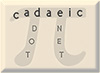All Small Connected Graphs

When working on a problem involving graphs recently, I needed a comprehensive visual list of all the (non-isomorphic) connected graphs on small numbers of nodes, and was surprised to find a dearth of such images on the web.  So I made some.  Below are images of the connected graphs from 2 to 7 nodes.  In the upper left of each box is a cardinal number (starting from 1) which can be used as a unique identifier for each graph having n nodes.  The number in the upper right of each box is the number of edges and the string of numbers at the bottom of the box gives the degree of each node from node 0 to node n-1, in order.

These pictures were created by starting from Brendan McKay's very useful data sets, processing these with showg (found here) into a more convenient format, sorting them within each n by increasing number of edges, and then converting into images.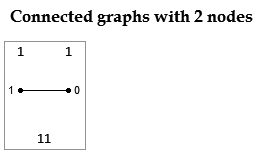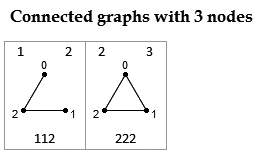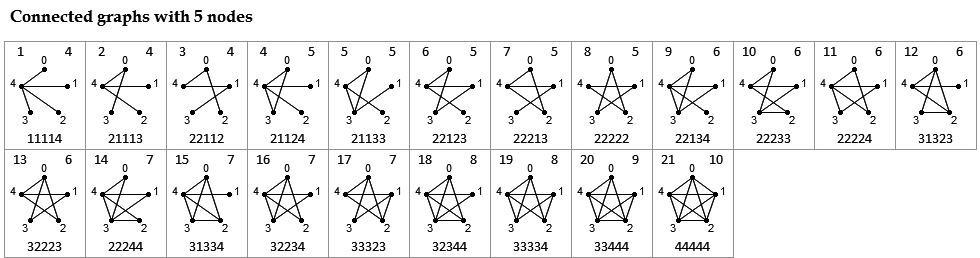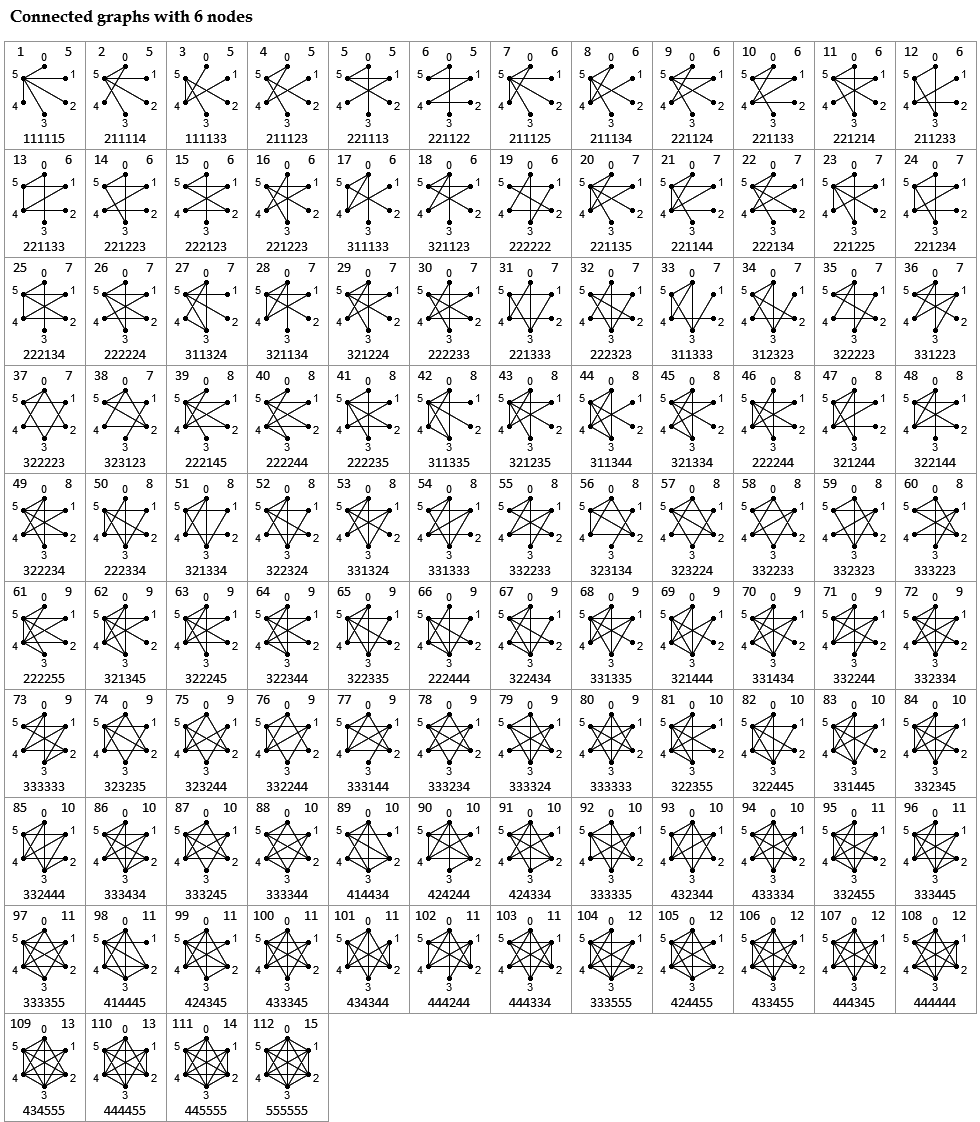For the 853 connected graphs with 7 nodes, go here.